#### 期刊菜单

Research on Optimizing Internal Control Structure of Eight Strand Tundish
DOI: 10.12677/MEng.2022.94039, PDF , HTML, XML, 下载: 93  浏览: 158  科研立项经费支持

Abstract: According to the principle of similarity, taking the eight strand tundish of a factory as the research object, the physical simulation study was carried out on the influence of different flow control element layout structures on the flow field in the tundish. Then, an industrial test was carried out in a steel factory, and 34 samples of the same steel grade for 6 casting times were sampled to analyze the inclusion rating in the steel before and after improvement. The results show that the flow field structure of the tundish with turbulence controller and L-shaped upward opening retaining wall is optimal, and the inclusions of type B and Ds in the steel of the improved scheme are significantly reduced, with their occurrence proportions reduced by 62.5% and 54.5% respectively, and the average level of inclusions reduced by 74.3% and 65.7% respectively. The improved scheme has obvious removal effect on inclusions in tundish.

1. 引言

2. 中间包物理模拟方案

2.1. 中间包物理模拟原理

${u}_{m}={\lambda }^{0.5}{u}_{p}$ (1)

${Q}_{m}={\lambda }^{2.5}{Q}_{p}$ (2)

${t}_{m}={\lambda }^{0.5}{t}_{p}$ (3)

2.2. 实验设备及RTD曲线分析

2.2.1. 实验设备

2.2.2. RTD曲线分析

$y=0.000666691x-0.0000850526$ (4)

1) 计算理论平均停留时间τ

$\tau =\frac{V}{Q}$ (5)

2) 时间的无量纲处理

${\theta }_{i}=\frac{{t}_{i}}{\tau }$ (6)

3) 浓度的无量纲处理

$E\left({\theta }_{i}\right)=\frac{c\left({t}_{i}\right)}{{\int }_{0}^{\infty }c\left({t}_{i}\right)\text{d}t}\tau$ (7)

4) 实际平均停留时间tf

${t}_{f}=\frac{{\int }_{0}^{\infty }\left[c\left({t}_{i}\right)×t\right]\text{d}t}{{\int }_{0}^{\infty }c\left({t}_{i}\right)\text{d}t}\tau$ (8)

5) 最短停留时间tmin

6) 峰值时间tmax

7) 活塞区体积分数 ${\theta }_{p}$

${\theta }_{p}=\frac{{V}_{p}}{V}=\frac{{t}_{\text{min}}+{t}_{\text{max}}}{2\tau }$ (9)

${\theta }_{d}=\frac{{V}_{d}}{V}=1-\frac{{\int }_{0}^{2\tau }\left[c\left({t}_{i}\right)×{t}_{i}\right]\text{d}t}{\tau ×{\int }_{0}^{2\tau }c\left({t}_{i}\right)\text{d}t}×{\int }_{0}^{2}E\left({\theta }_{i}\right)\text{d}\left(\theta \right)$ (10)

${\theta }_{m}=\frac{{V}_{m}}{V}=1-{\theta }_{p}-{\theta }_{d}$ (11)

(10)对于单入口多出口中间包的总体RTD曲线来说，在各流流量相同的前提下，取各时刻4流示踪剂浓度的算术平均值作为总体RTD曲线的浓度。

2.3. 中间包参数及实验方案

2.3.1. 中间包原型参数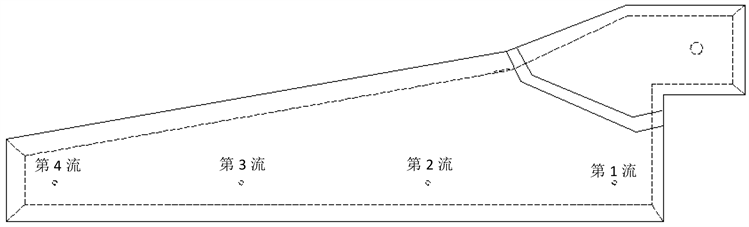Figure 1. Overall diagram of tundish original structure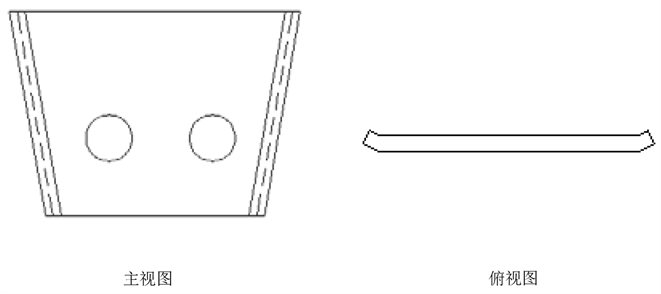Figure 2. Schematic diagram of original retaining wall structure

2.3.2. 中间包新内部结构参数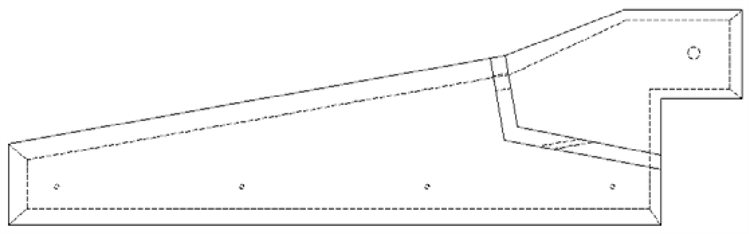Figure 3. Schematic diagram of internal structure of tundish new retaining wall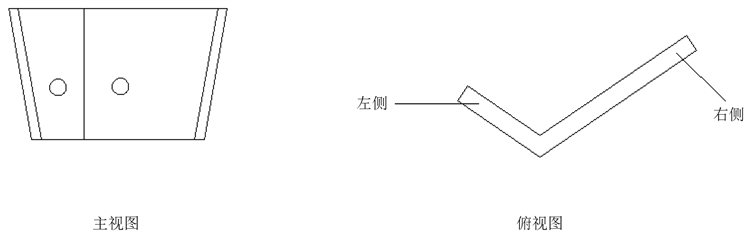Figure 4. Schematic diagram of new retaining wall structure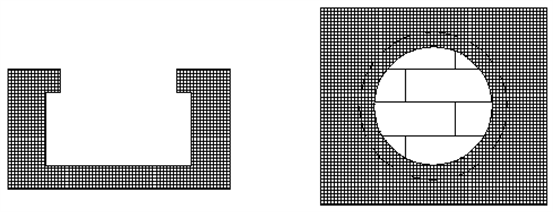Figure 5. Structure diagram of turbulence controller

2.3.3. 物理模拟实验方案

3. 物理模拟实验结果

3.1. 不同挡墙结构对中间包内流场特征的影响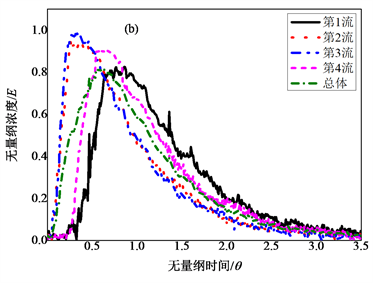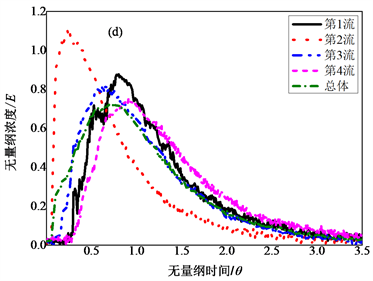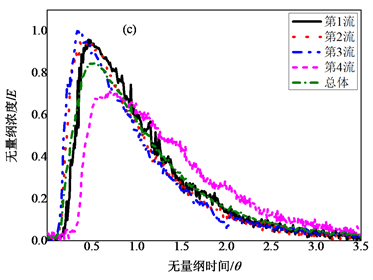Figure 6. Each flow and overall RTD curve of different schemes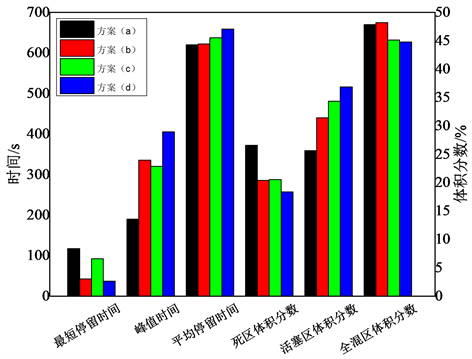Figure 7. Overall RTD curve characteristic parameters of different schemes and proportion of each region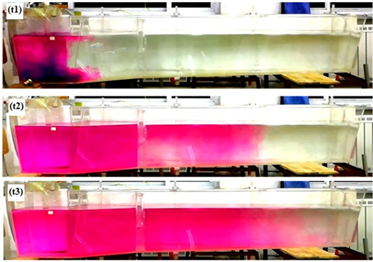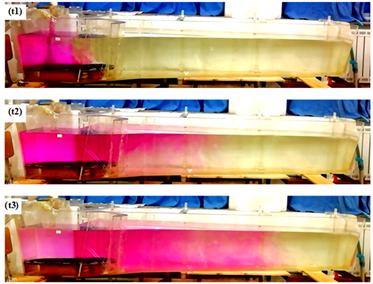方案(a) 方案(c)

Figure 8. Flow field diagram of tundish experiment process (t1) 3 s, (t2) 50 s, (t3) 120 s

3.2. 加湍流控制器实验对比Table 2. Flow field diagram of tundish test process

4. 工业试验对比Table 3. Data statistics of slab inclusions

5. 结论

1) 根据相似原理，建立中间包物理模型。在实验室中对不同结构挡墙进行实验，并将得到RTD曲线进行计算分析，综合各个特征参数可以看出，L型向上开孔的挡墙的流场形式最优；

2) 针对湍流控制器对中间包的影响，进行实验室实验，研究发现加入湍流控制器后中间包流场优于无湍流控制器中间包流场，因此L型向上开孔挡墙加湍流控制器为八流中间包的最优内控结构；

3) 在工业试验中，将最优内控结构与原挡墙进行对比试验，共取样34个，并将样品进行夹杂物检测，经统计分析可知采用最优内控结构铸坯中B类与Ds类夹杂在出现占比以及平均级别两方面相比于原挡墙均有明显的数量下降，达到了优化中间包内部结构净化钢水的目的。

NOTES

*通讯作者。

  刘逸波, 杨健. 中间包流场控制技术的进展[J]. 连铸, 2021(5): 12-33.  翟晓毅, 颜慧成, 杨海滨, 等. 八流中间包结构优化水模型试验研究[J]. 炼钢, 2018, 34(1): 25-29.  吴金强, 杨树峰, 李京社, 等. 三流中间包结构优化物理模拟[J]. 中国冶金, 2019, 29(11): 39-44.  李怡宏, 包燕平, 赵立华, 等. 多流中间包导流孔对钢液流动轨迹的影响[J]. 钢铁, 2014, 49(6): 37.  唐德池, 刘国梁, 马文俊, 等. 控流装置对中间包钢水流场和洁净度的影响[J]. 连铸, 2021(5): 103-107.  林路, 李翔, 包燕平. 单流中间包结构优化及工业试验[J]. 炼钢, 2017, 33(6): 30-36.  于洋, 岳峰, 肖波. 对称四流中间包结构优化[J]. 钢铁研究, 2017, 45(2): 15-18.  杨凌志, 朱荣, 李超, 林腾昌, 吴兵, 梁建国. 基于水力学模拟试验的3流连铸20 t中间包结构优化研究[J]. 特殊钢, 2015, 36(1): 1-4.  Sahai, Y. and Emi, T. (1996) Melt Flow Characterization in Continuous Casting Tundishes. ISIJ International, 36, 667. https://doi.org/10.2355/isijinternational.36.667  雷洪, 赵岩, 鲍家琳, 刘承军, 陈海耿, 赫冀成. 多流连铸中间包停留时间分布曲线总体分析方法[J]. 金属学报, 2010, 46(9): 1109-1114.  郑淑国, 朱苗勇. 多流连铸中间包内钢液流动特性的分析模型[J]. 金属学报, 2005(10): 67-70.